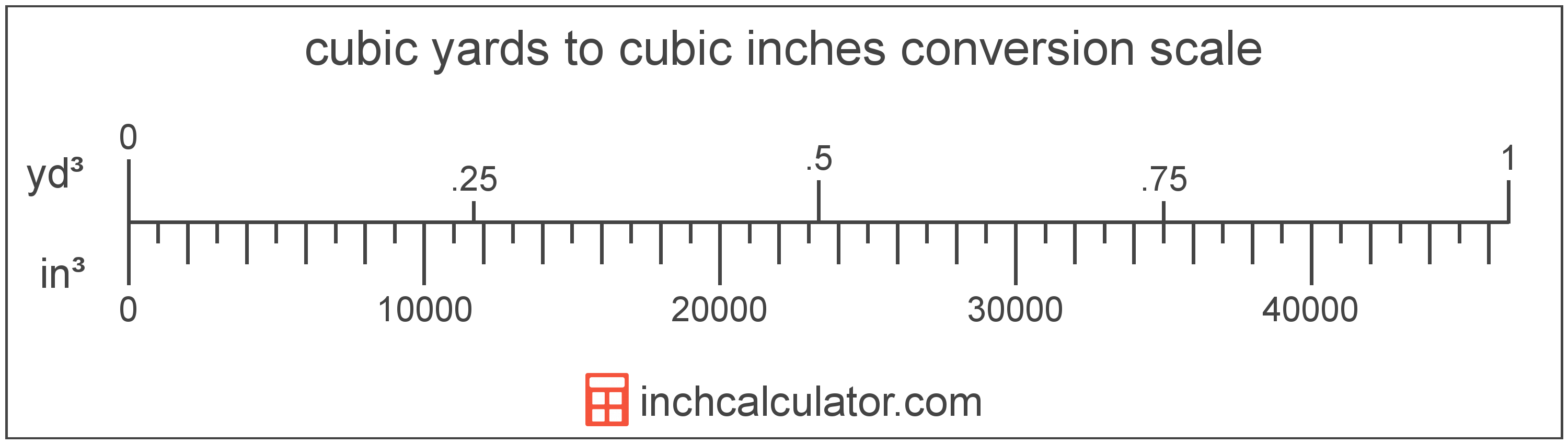# Cubic Inches to Cubic Yards Conversion

Enter the volume in cubic inches below to get the value converted to cubic yards.

Results in Cubic Yards:1 in³ = 2.1433E-5 yd³
Hint: use a scientific notation calculator to convert E notation to decimal

## How to Convert Cubic Inches to Cubic YardsTo convert a cubic inch measurement to a cubic yard measurement, multiply the volume by the conversion ratio.

Since one cubic inch is equal to 2.1433E-5 cubic yards, you can use this simple formula to convert:

cubic yards = cubic inches × 2.1433E-5

The volume in cubic yards is equal to the cubic inches multiplied by 2.1433E-5.

For example, here's how to convert 50,000 cubic inches to cubic yards using the formula above.
50,000 in³ = (50,000 × 2.1433E-5) = 1.071674 yd³

## Cubic Inches

A cubic inch is a unit of volume equal to the space consumed by a cube with sides that are one inch in all directions. One cubic inch is equivalent to about 16.387 cubic centimeters or 0.554 fluid ounces.

The cubic inch is a US customary and imperial unit of volume. A cubic inch is sometimes also referred to as a cubic in. Cubic inches can be abbreviated as in³, and are also sometimes abbreviated as cu inch, cu in, or CI. For example, 1 cubic inch can be written as 1 in³, 1 cu inch, 1 cu in, or 1 CI.

## Cubic Yards

The cubic yard is a unit of volume that is equal to the space consumed by a cube with each edge measuring one yard.

The cubic yard is a US customary and imperial unit of volume. A cubic yard is sometimes also referred to as a cubic yd. Cubic yards can be abbreviated as yd³, and are also sometimes abbreviated as cu yard, cu yd, or CY. For example, 1 cubic yard can be written as 1 yd³, 1 cu yard, 1 cu yd, or 1 CY.

You might also be interested in our cubic yards calculator.

## Cubic Inch to Cubic Yard Conversion Table

Cubic inch measurements converted to cubic yards
Cubic Inches Cubic Yards
1 in³ 0.000021433 yd³
2 in³ 0.000042867 yd³
3 in³ 0.0000643 yd³
4 in³ 0.000085734 yd³
5 in³ 0.000107 yd³
6 in³ 0.000129 yd³
7 in³ 0.00015 yd³
8 in³ 0.000171 yd³
9 in³ 0.000193 yd³
10 in³ 0.000214 yd³
100 in³ 0.002143 yd³
1,000 in³ 0.021433 yd³
10,000 in³ 0.214335 yd³
100,000 in³ 2.1433 yd³

## References

1. National Institute of Standards and Technology, Specifications, Tolerances, and Other Technical Requirements for Weighing and Measuring Devices, Handbook 44 - 2019 Edition, https://nvlpubs.nist.gov/nistpubs/hb/2019/NIST.HB.44-2019.pdf